# Random Variables vs. Algebraic Variables

beginning of content:

## The Challenge for Students

Most students are familiar with variables because they’re used in algebra. Random variables, however, differ from these algebraic variables in important ways that often bewilder students.

A random variable is often introduced to students as a value that is created by some random process. To get off to a good start, use props students are familiar with. Give students roll dice, flip coins, or draw cards so you can get the idea of a random variable across. However, you need to get students to see that the term “random variable” is used in both a more abstract way and a more varied way in most statistics textbooks. At times, it is used to refer to the outcome of a single event, as in P(X = k). At other times, it is used to refer to the entire pattern of possible outcomes rather than a single event (as in µx = 10). This concept may not be readily grasped by many students.

This contrasts with what they know about algebra, where variables typically have a single, though hidden, value. Finding this hidden value may stump students, to be sure, but they know there’s a value to be found. It’s true that sometimes in algebra, variables are used to refer to a pattern of many values. For example in the equation of a parabola, y = x2 + 3x - 4. However, a chief characteristic of a function is that for a given x value, y has only one possible value. Indeed, where variables are used as functions, a student solves the algebra problem by finding a particular value, or perhaps several values, such as the coordinates of the vertex or the intercepts.

## Illustrating the Difference

A random variable, on the other hand, is something completely different. Here’s a hypothetical that illustrates this difference:

Imagine that a teacher has an extra-credit scheme in which each student who completes all of a week’s assignments on time gets to roll a die, with bonus points equal to the result of the die added to the student’s score. At the beginning of the term, students enthusiastically respond by doing all their assignments, earning between 1 and 6 extra credit points. This point value, call it X, is a random variable because its value is determined by the outcome of a random process. There are six different possible values for X, the integers from 1 to 6. Students might think of the random variable X as representing a single, unknown value, in the same way that they think about algebraic variables. But X really refers to the distribution of possible values and the associated probabilities.

These are shown in Figure 1 below:

### Figure 1:

X 1 2 3 4 5 6
P(x) 1/6 1/6 1/6 1/6 1/6 1/6
The table has two rows. The top row shows the random variable for each of the 6 possible credit points: X = 1, 2, 3, 4, 5, and 6. The second row beneath shows the probability for each credit point P(x) is one/sixth for each variable above.

Using standard formulas, students encounter expressions like µx = 3.5 and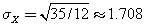, which use X in this way. Without much experience with these types of variables, it often takes some time for students to figure out what the various symbols actually represent.

Back to our hypothetical: As the semester wears on, some students are not completing all the work. So, as an extra incentive late in the term, the teacher decides to offer a double bonus, between 2 and 12 points. There are now two different ways that the teacher can proceed: Either have the student roll one die, then double the value, or roll two dice, and add the sum as bonus points.

Are these procedures any different from each other? They have the same maximum and minimum values (2 and 12) and the same mean (7). However, they neither have the same probability distribution nor the same variability. In the first case, there are only six possible outcomes when doubling the value of one die: the even numbers from 2 to 12. In the second case, all the values from 2 to 12 might result from the random process.

To examine the variability, we need to compare the probability distributions of each process. If X refers to the random variable of a single toss of a die, then the value of the random bonus in the first process, doubling the value of the roll, is 2X. Let’s call this new random variable D for “doubling.” We could write, correctly, D = 2X.

Here’s the probability distribution of D:

### Figure 2:

D 2 4 6 8 10 12
P(D) 1/6 1/6 1/6 1/6 1/6 1/6
The table has two rows. The top row shows the doubling of the random variable for each of the 6 possible credit points as: D = 2, 4, 6, 8, 10, and 12. The second row beneath shows the probability for each credit point P(D) remains one/sixth for each variable above.

The outcomes are uniformly distributed because all outcomes have the same probability of occurring. Again, employing the standard formulas µD = 7 and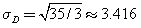, how do these compare to the values for rolling a single die, X?

It’s easy enough to see from the numerical values that µD = 2µX and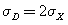, and these values are predicted by the rules for multiplying a random variable by a constant.

When two dice are rolled, though, the results are different. Call the random variable that represents the outcomes of the two-dice process T (for “two”). We could write T = X + X. This equation represents the fact that T is the result of two independent instances of the random variable T. Each time you write the symbol T, you imply a random draw from the specified population.

Here’s the probability distribution of T:

### Figure 3:

T 2 3 4 5 6 7 8 9 10 11 12
P(T) 1/36 2/36 3/36 4/36 5/36 6/36 5/36 4/36 3/36 2/36 1/36
The table has two rows. The top row shows the random variable for T as: T = 2, 3, 4, 5, 6, 7, 8, 99, 10, 11, and 12. The second row beneath shows the probability P(T) as one/thirty sixth, two/thirty sixths, three/thirty sixths, four/thirty sixths, five/thirty sixths, six/thirty sixths, five/thirty sixths, four/thirty sixths, three/thirty sixths, two/thirty sixths, and one/thirty sixth for each variable above.

Again employing the standard formulas µT = 7 and, since (T) results from two independent instances of (X), the formulas for calculating the mean and variance of independent random variables can be used to confirm the values for the mean and standard deviation of (T). So we have µT = µX + µX = 2µX = 7 and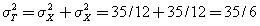, and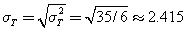.

It’s clear from these values and the discussion above that (T) ≠ (D)open parenthesis T close parenthesis not equal to open parenthesis D close parenthesis, or (X) + (X) ≠ 2(x)open parenthesis X close parenthesis plus open parenthesis X close parenthesis not equal to 2 open parenthesis x close parenthesis. This is a little hard for many students to absorb and is part of the difficulty they face sorting out the differences.

Students might also have difficulty with the term “variability” in the context of random variables. At first glance, many students would describe the second process as more variable. It can result in 11 different outcomes, as opposed to only six in the first case. They mistake the idea of variety for the more formally defined measures of variability.

As you introduce your students to random variables, be ready to clear up confusion regarding ideas they are accustomed to, algebraic variables, and the new ideas. Provide your students with settings in which they can work with random variables, write expressions using random variables, and gain the intuition that they need if they are to use these ideas effectively in their work in statistics.

## Authored by

• Peter Flanagan-Hyde
Phoenix Country Day School